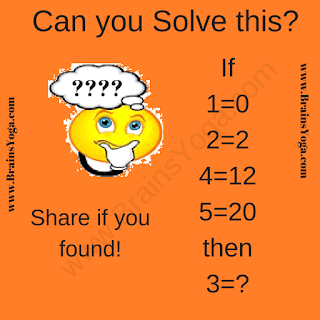## Saturday, April 28, 2018

Here is one hard logical reasoning puzzle which will twist your brain. In this logical reasoning puzzle, your challenge is decode the logic of the equations and then solve the last equation to find the value of missing number which will replace the question mark. So are you ready to crack this logical reasoning puzzle?Hard Logical Reasoning Puzzle for Teens

Answer of this "Hard Logical Reasoning Puzzle for Teens", can be viewed by clicking on button. Please do give your best try before looking at the answer.

#### 1 comment:

1.Answer is 6. If A=B is the given Mathematical equation then the logical expression which relates numbers in these equations is A*(A-1) = B